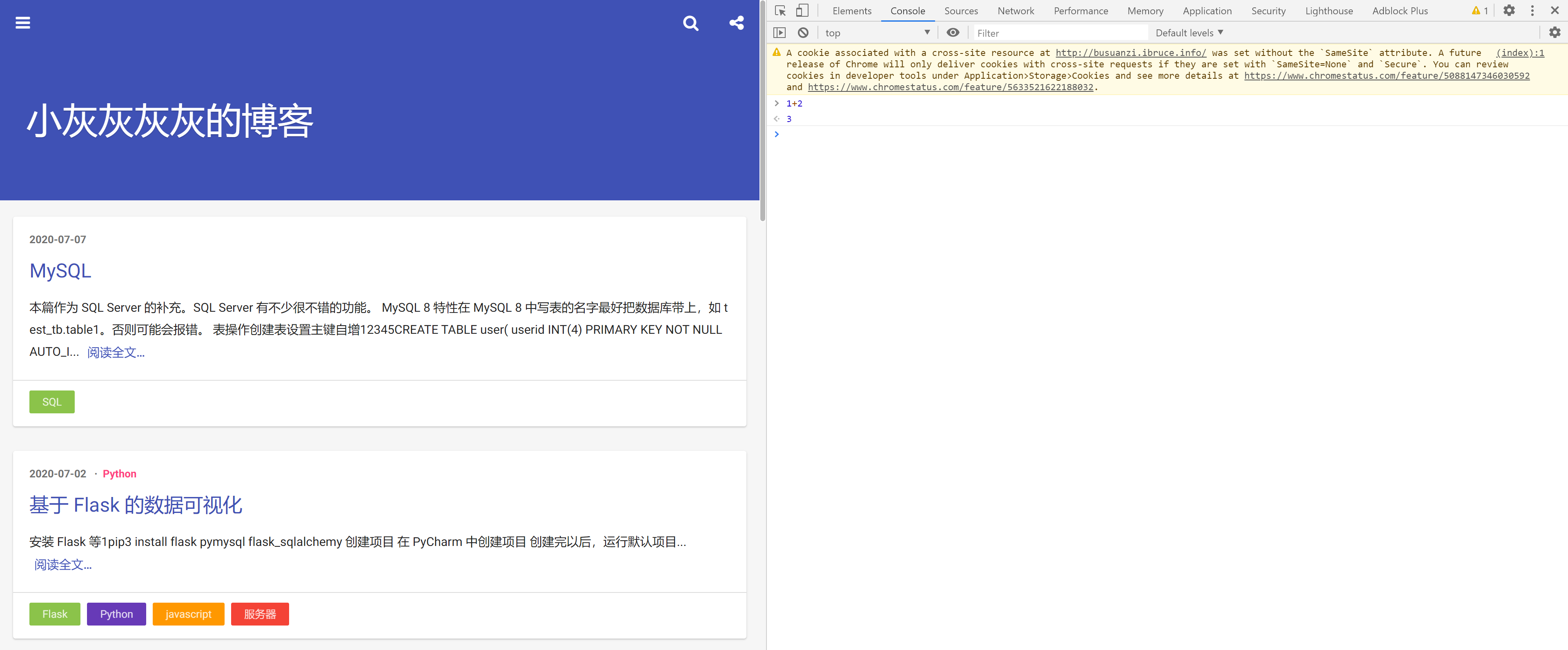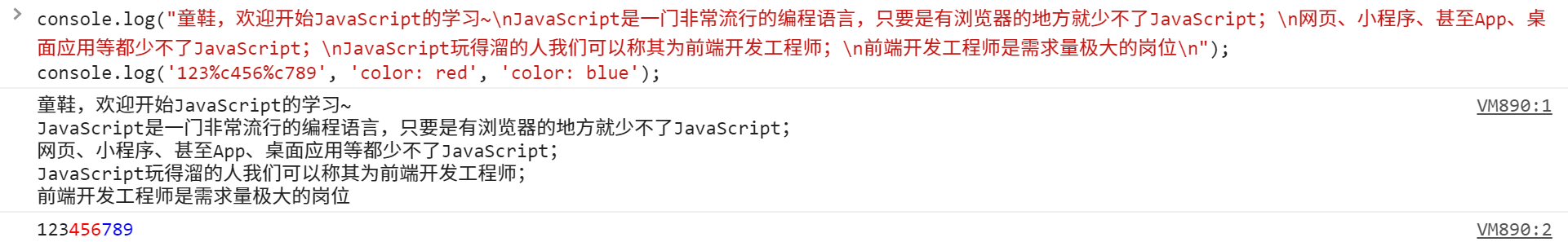## # 运行 JavaScript## # JavaScript 语法基础

• 行尾需要 `;`
• 注释为 `//`
• 字符串和 Python 相同，可用单/双引号，可使用 `\n` `\\` 等扩展
• 函数名推荐使用驼峰法来命名 `camelCase`

## # 打印输出

``````console.log("童鞋，欢迎开始JavaScript的学习~\nJavaScript是一门非常流行的编程语言，只要是有浏览器的地方就少不了JavaScript；\n网页、小程序、甚至App、桌面应用等都少不了JavaScript；\nJavaScript玩得溜的人我们可以称其为前端开发工程师；\n前端开发工程师是需求量极大的岗位\n");
console.log('123%c456%c789', 'color: red', 'color: blue');
``````## # 数据

### # 数学运算

``````136+384; //加法
(110/0.5+537-100)*2; //加减乘除
2**5; //指数运算符
``````

### # 声明 let

`let` 用于声明变量。在脚本中，变量不能被声明第二次（调试窗口是可以的）。

``````let a;     // 声明
a = 1;     // 赋值
let b = 2; // 声明并赋值
let a;     // 重复声明，报错
b = "123"; // 重新赋值为新的类型，允许
``````

### # 常量 const

``````const window_weight = 3840;
const window_height = 2160;
``````

## # 数组

``````movielist=["肖申克的救赎","霸王别姬","这个杀手不太冷","阿甘正传","美丽人生"];
Console.log(movielist); // "这个杀手不太冷"
``````

## # 流程控制

### # for

``````let str = '';

for (let i = 0; i < 9; i++) {
str = str + i;
}

console.log(str);
// expected output: "012345678"
``````
``````const array1 = ['a', 'b', 'c'];

array1.forEach(element => console.log(element));

// expected output: "a"
// expected output: "b"
// expected output: "c"
``````

``````a = [9,8,7];

for (let i of a)
console.log(i)
// 9
// 8
// 7

for (let i in a)
console.log(i)
// 0
// 1
// 2
``````
``````b = {c:1, d:2};

for (let i of b)
console.log(i)
// Uncaught TypeError: b is not iterable

for (let i in b)
console.log(i)
// c
// d
``````

## # 常用函数

• `join()` 将数组按指定分隔符拼接为字符串；
• `push()` 在数组末尾追加元素（返回值为新数组的长度）；
• `pop()` 返回并移除最后一个元素；

• `concat()` 返回由当前数组和其他数组/元素组合而成的新数组；
• `reverse()`
• `shift()` 返回并移除第一个元素；
• `slice()` 抽取当前数组中的一段元素组合成一个新数组，即 Python 的切片；
• `sort()` 原地排序数组；
• `splice()`
• `unshift()` 在数组开头追加元素（返回值为新数组长）；

## # 对象

``````movie={
name: "霸王别姬",
image: "https://img3.doubanio.com/view/photo/s_ratio_poster/public/p1910813120.webp",
desc: "风华绝代。"
}
``````

``````//两种方法都行
console.log(move.name);
console.log(movie["name"]);
``````

``````movie.englishname = "Farewell My Concubine"; // 添加属性
delete movie.img;                            // 删除属性
movie.desc = "人生如戏。";                    // 修改属性
``````

## # 函数与调用函数

``````function square(number) {
return number * number;
};
square(5);

// 匿名函数
let square = function(number) {
return number * number
};
square(5)

// 箭头函数（Arrow Function）
const multiply = (x, y) => {
return x * y;
}
const sum= (x, y) => x + y;// 连{}和return语句都可以省掉
console.log(multiply(20, 4));
console.log(sum(20, 4));

// JavaScript 函数
scrollToPosition() {
},
yellowTap:function(){
},
``````

``````// 匿名函数（定义在 Vue 中的 methods）
// 输出 Vue {_uid: 0, _isVue: true, \$options: {…}, _renderProxy: Proxy, _self: Vue, …}
console.warn(this)
}

// 箭头函数（定义在 Vue 中的 methods）
// 输出 Window {window: Window, self: Window, document: document, name: "", location: Location, …}
console.warn(this)
}
``````

## # 调用其他文件的函数

`date.js` 中这样写道：

``````// date.js
export function getDate() {
// function body
}
``````

``````import {getDate} from '/utils/date.js';

getDate(); // 即可调用
``````

``````// date.js
export default function () {
// function body
}
``````

``````import getDate23333 from '/utils/date.js'; // 可自定义函数名

getDate23333(); // 即可调用
``````